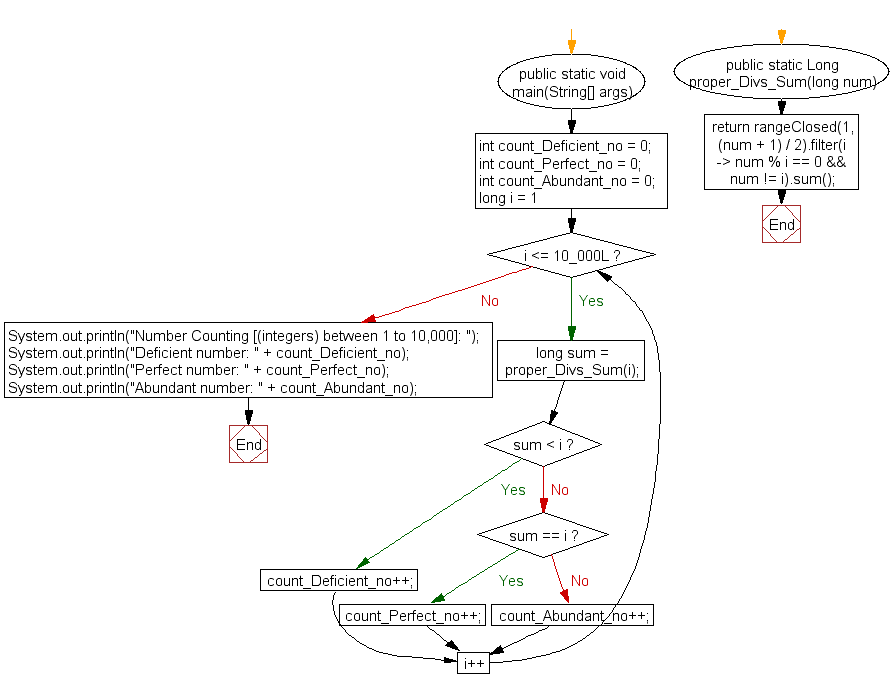﻿ Java exercises: Classify Abundant, deficient and perfect number (integers) between 1 to 10,000 - w3resource# Java Exercises: Classify Abundant, deficient and perfect number (integers) between 1 to 10,000

## Java Numbers: Exercise-2 with Solution

Write a Java program to classify Abundant, deficient and perfect number (integers) between 1 to 10,000.

In number theory, an abundant number is a number for which the sum of its proper divisors is greater than the number itself.
Example :
The first few abundant numbers are:
12, 18, 20, 24, 30, 36, 40, 42, 48, 54, 56, 60, 66, 70, 72, 78, 80, 84, 88, 90, 96, 100, 102,…
The integer 12 is the first abundant number. Its proper divisors are 1, 2, 3, 4 and 6 for a total of 16.
Deficient number: In number theory, a deficient number is a number n for which the sum of divisors σ(n)<2n, or, equivalently, the sum of proper divisors (or aliquot sum) s(n)<n. The value 2n − σ(n) (or n − s(n)) is called the number's deficiency.
As an example, divisors of 21 are 1, 3 and 7, and their sum is 11. Because 11 is less than 21, the number 21 is deficient. Its deficiency is 2 × 21 − 32 = 10.
The first few deficient numbers are:
1, 2, 3, 4, 5, 7, 8, 9, 10, 11, 13, 14, 15, 16, 17, 19, 21, 22, 23, 25, 26, 27, 29, 31, 32, 33, …….
Perfect number: In number system, a perfect number is a positive integer that is equal to the sum of its proper positive divisors, that is, the sum of its positive divisors excluding the number itself.
Equivalently, a perfect number is a number that is half the sum of all of its positive divisors (including itself) i.e. σ1(n) = 2n.
The first perfect number is 6. Its proper divisors are 1, 2, and 3, and 1 + 2 + 3 = 6. Equivalently, the number 6 is equal to half the sum of all its positive divisors: ( 1 + 2 + 3 + 6 ) / 2 = 6. The next perfect number is 28 = 1 + 2 + 4 + 7 + 14. This is followed by the perfect numbers 496 and 8128.

Pictorial Presentation:Sample Solution:

Java Code:

``````import static java.util.stream.LongStream.rangeClosed;
public class Exercise2 {

public static void main(String[] args) {
int count_Deficient_no = 0;
int count_Perfect_no = 0;
int count_Abundant_no = 0;

for (long i = 1; i <= 10_000L; i++) {
long sum = proper_Divs_Sum(i);
if (sum < i)
count_Deficient_no++;
else if (sum == i)
count_Perfect_no++;
else
count_Abundant_no++;
}
System.out.println("Number Counting [(integers) between 1 to 10,000]: ");
System.out.println("Deficient number: " + count_Deficient_no);
System.out.println("Perfect number: " + count_Perfect_no);
System.out.println("Abundant number: " + count_Abundant_no);
}
public static Long proper_Divs_Sum(long num) {
return rangeClosed(1, (num + 1) / 2).filter(i -> num % i == 0 && num != i).sum();
}
}
```
```

Sample Output:

```Number Counting [(integers) between 1 to 10,000]:
Deficient number: 7508
Perfect number: 4
Abundant number: 2488
```

Flowchart:Java Code Editor:

What is the difficulty level of this exercise?

﻿

## Java: Tips of the Day

Array vs ArrayLists:

The main difference between these two is that an Array is of fixed size so once you have created an Array you cannot change it but the ArrayList is not of fixed size. You can create instances of ArrayLists without specifying its size. So if you create such instances of an ArrayList without specifying its size Java will create an instance of an ArrayList of default size.

Once an ArrayList is full it re-sizes itself. In fact, an ArrayList is internally supported by an array. So when an ArrayList is resized it will slow down its performance a bit as the contents of the old Array must be copied to a new Array.

At the same time, it's compulsory to specify the size of an Array directly or indirectly while creating it. And also Arrays can store both primitives and objects while ArrayLists only can store objects.

Ref: https://bit.ly/3o8L2KH